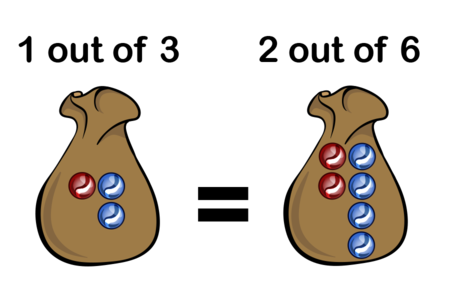# Odds: Introduction

Lets say we have a bag containing twice as many blue marbles as red marbles. Then, if you reach in without looking and pick out a marble at random, the odds are 2 : 1 in favor of drawing a blue marble as opposed to a red one.

Odds express relative quantities. 2 : 1 odds are the same as 4 : 2 odds are the same as 600 : 300 odds. For example, if the bag contains 1 red marble and 2 blue marbles, or 2 red marbles and 4 blue marbles, then your chance of pulling out a red marble is the same in both cases:In other words, given odds of $$(x : y)$$ we can scale it by any positive number $$\alpha$$ to get equivalent odds of $$(\alpha x : \alpha y).$$

# Converting odds to probabilities

If there were also green marbles, the relative odds for red versus blue would still be (1 : 2), but the probability of drawing a red marble would be lower.If red, blue, and green are the only kinds of marbles in the bag, then we can turn odds of $$(r : b : g)$$ into probabilities $$(p_r : p_b : p_g)$$ that say the probability of drawing each kind of marble. Because red, blue, and green are the only possibilities, $$p_r + p_g + p_b$$ must equal 1, so $$(p_r : p_b : p_g)$$ must be odds equivalent to $$(r : b : g)$$ but “normalized” such that it sums to one. For example, $$(1 : 2 : 1)$$ would normalize to $$\frac{1}{4} : \frac{2}{4} : \frac{1}{4},$$ which are the probabilities of drawing a red /​ blue /​ green marble (respectively) from the bag on the right above.

Note that if red and blue are not the only possibilities, then it doesn’t make sense to convert the odds $$(r : b)$$ of red vs blue into a probability. For example, if there are 100 green marbles, one red marble, and two blue marbles, then the odds of red vs blue are 1 : 2, but the probability of drawing a red marble is much lower than 1/​3! Odds can only be converted into probabilities if its terms are mutually exclusive and exhaustive.

Imagine a forest with some sick trees and some healthy trees, where the odds of a tree being sick (as opposed to heathy) are (2 : 3), and every tree is either sick or healthy (there are no in-between states). Then the probability of randomly picking a sick tree from among all trees is 2 /​ 5, because 2 out of every (2 + 3) trees is sick.In general, the operation we’re doing here is taking relative odds like $$(a : b : c \ldots)$$ and dividing each term by the sum $$(a + b + c \ldots)$$ to produce $$\left(\frac{a}{a + b + c \ldots} : \frac{b}{a + b + c \ldots} : \frac{c}{a + b + c \ldots}\ldots\right)$$\$ Dividing each term by the sum of all terms gives us an equivalent set of odds (because each element is divided by the same amount) whose terms sum to 1.

This process of dividing a set of odds by the sum of its terms to get a set of probabilities that sum to 1 is called normalization.

# Converting probabilities to odds

Let’s say we have two events R and B, which might be things like “I draw a red marble” and “I draw a blue marble.” Say $$\mathbb P(R) = \frac{1}{4}$$ and $$\mathbb P(B) = \frac{1}{2}.$$ What are the odds of R vs B?$$\mathbb P(R) : \mathbb P(B) = \left(\frac{1}{4} : \frac{1}{2}\right),$$ of course.

Equivalently, we can take the odds $$\left(\frac{\mathbb P(R)}{\mathbb P(B)} : 1\right)$$, because $$\frac{\mathbb P(R)}{\mathbb P(B)}$$ is how many more times likely R is than B. In this example, $$\frac{\mathbb P(R)}{\mathbb P(B)} = \frac{1}{2},$$ because R is half as likely as B. Sometimes, the quantity $$\frac{\mathbb P(R)}{\mathbb P(B)}$$ is called the “odds ratio of R vs B,” in which case it is understood that the odds for R vs B are $$\left(\frac{\mathbb P(R)}{\mathbb P(B)} : 1\right).$$

# Odds to ratios

When there are only two terms $$x$$ and $$y$$ in a set of odds, the odds can be written as a ratio $$\frac{x}{y}.$$ The odds ratio $$\frac{x}{y}$$ refers to the odds $$(x : y),$$ or, equivalently, $$\left(\frac{x}{y} : 1\right).$$

Parents:

• Odds

Odds express a relative probability.

• The $$x/y$$ notation is confusing—these ratios aren’t probabilities are they?